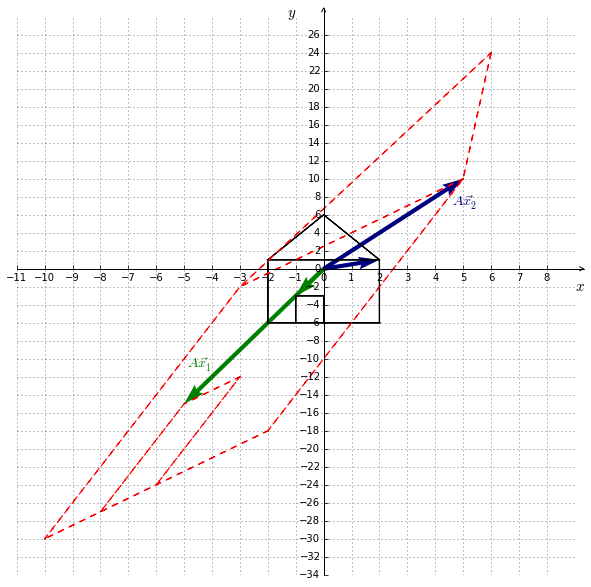## 1. Definition

We have seen that a matrix $A$ multiplying a vector $\vec{x}$ is in fact a linear transformation.

We now are interested in the vector $\vec{x}$ whose image  $\vec{y}$ (under the linear transformation) is linearly dependent to itself, namely :

$\lambda$ and $\vec{x}$ satisfying $(1)$ are called respectively eigenvalues and eigenvectors.

In $(1)$ $\boldsymbol{\vec{0}}$ is excluded. Indeed we know anyway that $\underbrace{A\,\vec{0}}_{ \vec{0} } = \underbrace{ \lambda\, \vec{0} }_{ \vec{0} } \,$ is true for any value of $\lambda$.

## 2. Calculation

From $(1)$ we can obtain :

In $(5)$, the multiplication matrix by vector of $(4)$ has been decomposed. The columns $\vec{C_1}, \dots, \vec{C_n}$ must be linearly dependent since the $x_i$ cannot equal zero because of $(1)$. Therefore their determinant equal zero.

Once the $\lambda$’s (eigenvalues) are found by solving the determinant, we replace them in the matrix in $(4)$ for determining the associated eigenvectors.

#### Example I

Let $h$ : $h \colon \, \mathbb{R}^2 \to \mathbb{R}^2$, $\vec{x} \mapsto \left( \begin{smallmatrix} 2 & 1 \\ 3 & 4 \end{smallmatrix} \right) \vec{x}$.
Let’s determine its eigenvalues and eigenvectors.

Based on $(4)$, we have :

The columns of the matrix in $(6)$ are linearly dependent, thus :

From $(7)$, we get : $\lambda_1 =1$ and $\lambda_2 =5$

To determine the eigenvector $\vec{x}_1$ associated to $\lambda_1$, we replace $\lambda_1$ in $(6)$ :

$\iff 1x+1y = 0 \iff x=-y$.  Let $x= \beta$.

To determine the eigenvector $\vec{x}_2$ associated to $\lambda_2$, we replace $\lambda_2$ in $(6)$ :

$\iff -3x+1y = 0 \iff y=3x \iff x=\frac{y}{3}$.  Let $x= \beta$.

## 3. But more concretely ?Figure 10.1 : eigenvector and an ordinary vector

Figure 10.1 shows a shape before and after the transformation (in dashed line) under the linear transformation of example I.

The eigenvector $\,\color{green}{\vec{x_1}}=\left(\begin{smallmatrix} -1 \\ -3 \end{smallmatrix}\right)$, as well as the ordinary vector   $\color{navy}{\vec{x_2}}=\left(\begin{smallmatrix} 2 \\ 1 \end{smallmatrix}\right)$ are represented too. The eigenvector does not rotate under the linear transformation.

## Recapitulation

An eigenvector is a vector whose image (under the linear transformation) is linearly dependent to itself, namely $\underbrace{ A\vec{x} }_{ \vec{y} } = \lambda \vec{x}$.

First the eigenvalues are found by solving the characteristic polynomial and after that the associated eigenvectors can be found.

The characteristic polynomial is obtained by computing the determinant of the matrix $A -\lambda I\,$.

By definition, an eigenvector does not equal to the null vector.